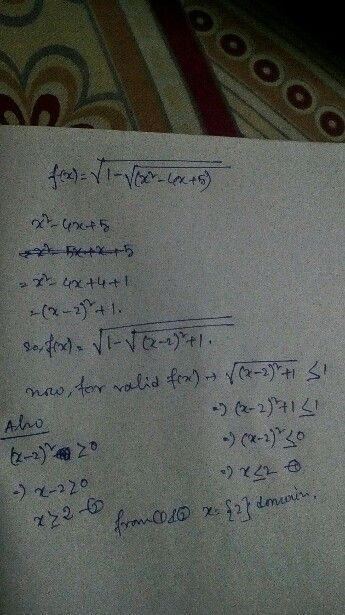Symbol
ProblemDomain of the function, $f\left(x\right)=\sqrt{1-\bar{\sqrt{\left(x^{2}} -4x+5\right)} }$ is $\left(1\right)$ $0$ $\left(2\right)$ $\left(3\right)$ $\left(2\right)$ $\left(4\right)$ $8-121\sqrt{}$ $1-\sqrt{1} ^{16}$